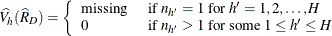#### Domain Statistics

When you use a DOMAIN statement to request a domain analysis, the procedure computes the requested statistics for each domain.

For a domain D, let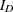be the corresponding indicator variable: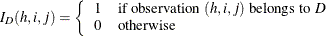Let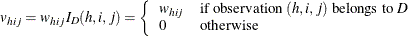The requested statistics for variable y in domain D are computed by using the new weights v.

##### Domain Mean

The estimated mean of y in the domain D is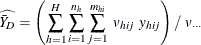where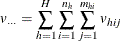The variance of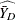is estimated by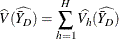where, if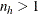, then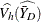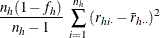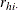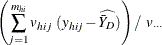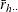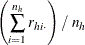and if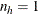, then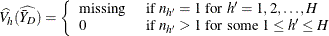##### Domain Total

The estimated total in domain D is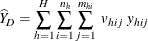and its estimated variance is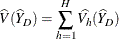where, if, then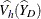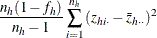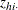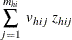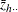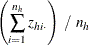and if, then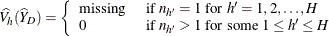##### Domain Ratio

The estimated ratio of Y to X in domain D is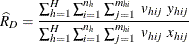and its estimated variance is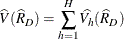where, if, then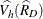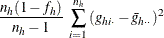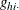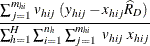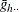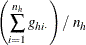and if, then